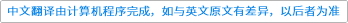Journal of Computer and System Sciences ( IF 1.494 ) Pub Date : 2021-01-08 , DOI: 10.1016/j.jcss.2020.12.004
Leszek Gąsieniec; Jesper Jansson; Christos Levcopoulos; Andrzej Lingas; Mia Persson

Henzinger et al. posed the so-called Online Boolean Matrix-vector Multiplication (OMv) conjecture and showed that it implies tight hardness results for several basic dynamic or partially dynamic problems [STOC'15]. We first show that the OMv conjecture is implied by a simple off-line conjecture that we call the MvP conjecture. We then show that if the definition of the OMv conjecture is generalized to allow individual (i.e., it might be different for different matrices) polynomial-time preprocessing of the input matrix, then we obtain another conjecture (called the OMvP conjecture) that is in fact equivalent to our MvP conjecture. On the other hand, we demonstrate that the OMv conjecture does not hold in restricted cases where the rows of the matrix or the input vectors are clustered, and develop new efficient randomized algorithms for such cases. Finally, we present applications of our algorithms to answering graph queries.Henzinger等。提出了所谓的在线布尔矩阵向量乘积（OMv）猜想，并表明对于几个基本的动态或部分动态问题，它暗示了严格的硬度结果[STOC'15]。我们首先表明OMv猜想是由简单的离线暗示的我们称之为MvP猜想。然后，我们证明，如果将OMv猜想的定义推广到允许对输入矩阵进行多项式时间预处理的单个（即，对于不同的矩阵可能有所不同），那么我们将得到另一个猜想（称为OMvP猜想）事实等同于我们的MvP猜想。另一方面，我们证明了在矩阵行或输入向量行聚集的受限情况下OMv猜想不成立，并针对此类情况开发了新的有效随机算法。最后，我们介绍了我们的算法在回答图查询中的应用。

down
wechat
bug